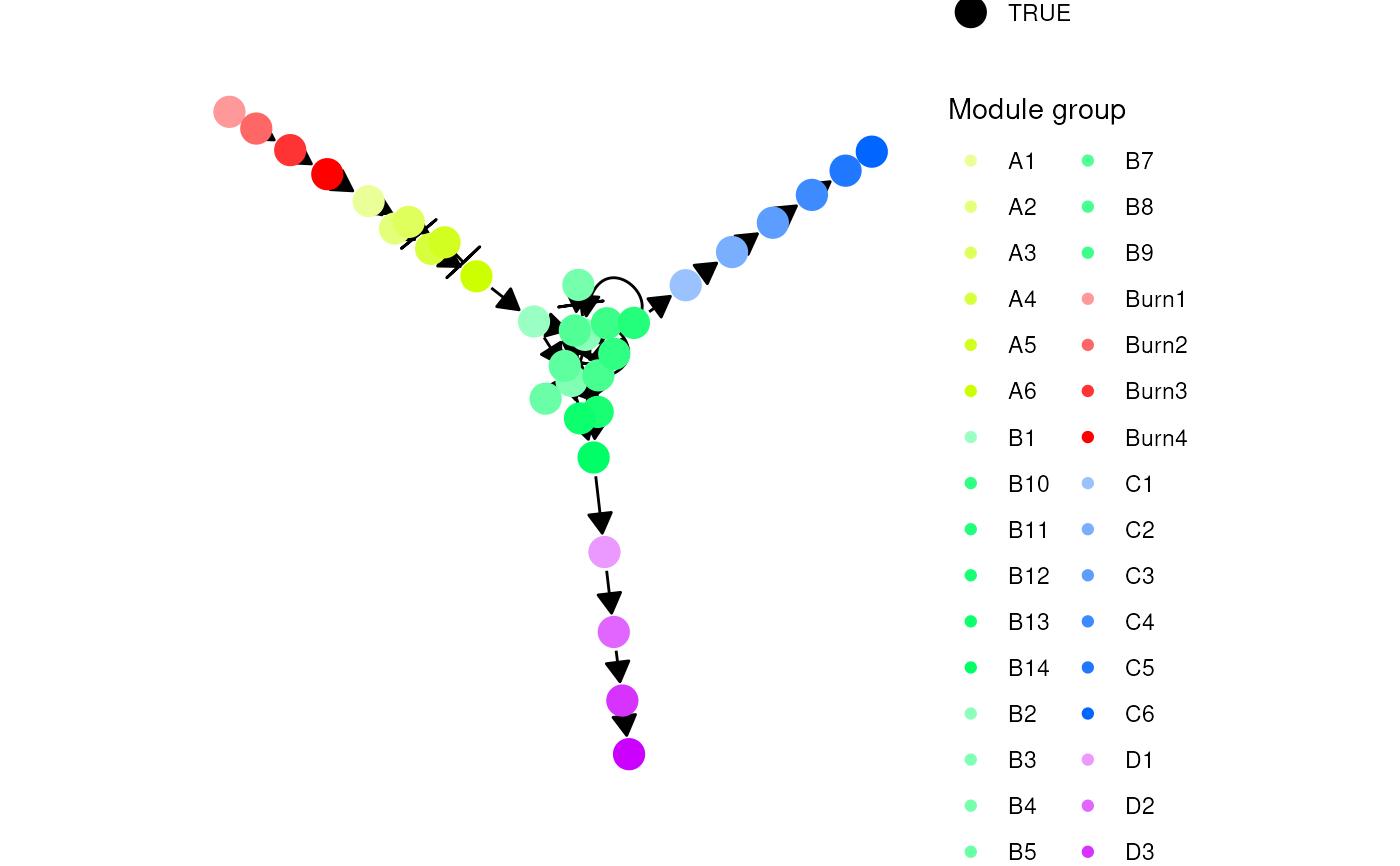generate_tf_network() generates the transcription factors (TFs) that drive the dynamic process a cell undergoes. tf_network_default() is used to configure parameters pertaining this process.

generate_tf_network(model)

tf_network_default(
min_tfs_per_module = 1L,
sample_num_regulators = function() 2,
weighted_sampling = FALSE
)

## Arguments

model A dyngen initial model created with initialise_model(). The number of TFs to generate per module in the backbone. A function to generate the number of TFs per module each TF will be regulated by. When determining what TFs another TF is regulated by, whether to perform weighted sampling (by rank) or not.

## Value

A dyngen model.

dyngen on how to run a complete dyngen simulation

## Examples

model <-
initialise_model(
backbone = backbone_bifurcating()
)
model <- model %>%
generate_tf_network()
#> Generating TF network
# \donttest{
plot_feature_network(model)# }